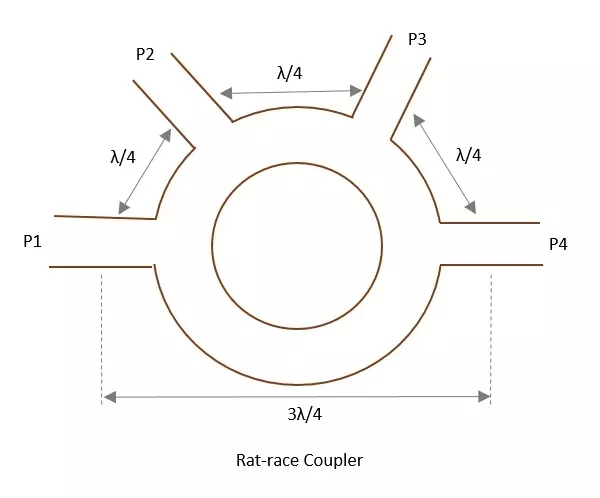# What is a rat-race junction in microwaves?

Rat-race junction is a microwave device used when there is a need to combine two signals with no phase difference and to avoid the signals that have a path difference.

A normal three port Tee junction is taken and 4th port is added to it, to make this rat-race junction. All of these ports are connected in angular ring forms at equal intervals using series or parallel junctions.

The mean circumference of total race be 1.5λ and that each of the four ports is separated by a distance of λ/4. The below figure shows the image of a Rat-race junction.Let us consider a few cases, to understand the operation of a Rat-race junction.

## Case1

If the input power is applied at port 1, it gets equally split into two ports, but in the clockwise direction for port2 and anti-clockwise direction for port 4. The port 3 has absolutely no output.

Why because, at ports 2 and 4, the powers combine in phase, whereas at port 3, cancellation occurs due to λ/2 path difference.

## Case 2

If the input power is applied at port 3, the power gets equally divided between port 2 and port 4. But there will be no output at port 1.

## Case 3

If two unequal signals are applied at port 1 itself, then the output will be proportional to the sum of the two input signals, which is divided between port 2 and 4. Now at port 3, the differential output appears.